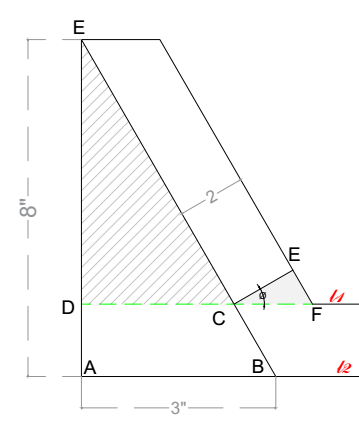# Show that the three triangles are similar

#### This is an additional solution for this problem. That is, to show that the created triangles from the given trapezoid are similar.In this problem, show that triangles BAE, CDE and FEC are all similar.

##### Solution:

One method to show this is to prove that corresponding angles of each triangle are equal.

Statement Reason
Triangles BAE, CDE and FEC
DC || AB Since DC and AB are extended (base)lines of a parallel trapezoids.
Angle A and angle D are both right angles Intersection of altitude and a horizontal line forms a right angle.
Angle C and angle B are congruent Parallel lines cut by a tranversal lines - corresponding angles.
Thus triangle BAE and CDE are similar AA or AAA theorem
Angle E is a right angle Line CE is the distance between two parallel lines.
∠ F + 90 + $$\phi$$ = 180 Sum of interior angles of a triangle is 180
∠ C + 90 + $$\phi$$ = 180 Supplementary angles
∠ F = ∠ C Simplifying the previous two equations
∠ D = ∠ = E = 90 All 90 are equal
Triangle FEC and CDE are similar AA theorem
The three triangles are similar Transitivity

** Use the given figure for clarity and other missing information.